﻿ Getting Started Tutorials - MechDesigner > Tutorial 18: Math FB > Step 18:0: Introduction to the Math-FB

# Step 18:0: Introduction to the Math-FB

### Math FB

IMPORTANT NOTES:

 Equations You must enter the equations correctly with the correct syntax. The Math FB will check the syntax, but not the equation itself. If you enter an incorrect equation, the output will be incorrect. For example, if you enter the equation  ((u*t) + (0.5*a*t))to find the final velocity of a point that has constant acceleration then the result will be incorrect. The correct equation is ((u*t) + (0.5*a*t*t). Note - a bad example as MechDesigner finds the velocity of all Points at all times for you. Motion Derivatives YOU must add the symbolic equation of each motion-derivative. If your application needs position, velocity, and acceleration, then you must enter the three equations separately. If you do not add the motion-derivatives (velocity and acceleration), their values remain at equal to the values at the input-connector. Units Use the Output Data Type drop-down box in the Math FB dialog-box  to select the units at the output-connector. The units inside the Math FB are S.I. units. For example, •50mm at the input-connector becomes 0.05m when applied to an equation inside the Math FB dialog-box •50° at the input-connector becomes (50*2*pi/360)rads. and applied to an equation inside the Math FB dialog-box

#### What can you do with a Math FB?

 The Math FB can use data that is available at the output-connectors of other FBs. You can use the Math FB to do: Simple Algebra For example: 2+3. The output will be 5, of course. Parametric Constants For example, connect the output of a Motion-Dimension FB of a Crank and the output of a Force-Data FB of the same Joint. You can use the Math FB to multiply the Torque(Nm) and the Velocity(rad/sec) values to give the Power(kW) as the output. Top-Tip: Connect a Gearing FB to the input of a Math FB. You can edit the Gearing FB, so that it becomes a parametric-constant of an equation in the Math FB, for example, a Friction Coefficient. Change the Units of a Variable For example, change the units at the input-connector of a Math FB to different units at its output-connector - for example: mm to Newtons.

#### To Add a Math FB, and Open the Math FB dialog-box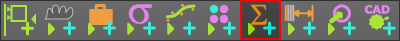1.Click modeling-FB toolbar > Math FB 2.Click the graphic-area

The Math FB is now in the graphic-area

Open a Math FB dialog-box

 1.Double-click the Math FB. or 1..

The Math FB dialog-box is now open

Math FB dialog-box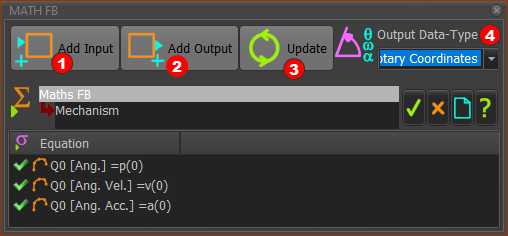Add Input buttonYou need one input-connector for each variable or parameter in your equation.

Add Output button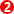You need one output-connector for each variable and its motion-derivative

Output Data-Type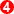1.Click the drop-down box to select the Data-Type for all of the output-connectors.

There is ONE Data-Type for All of the Output-Connectors.

Wire Numbers  and Data-Channels

The format of a variable in an equation is: Data-Channel (Wire-Number)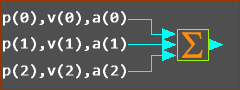Three wires connected to the input of a Math FB

The image above shows three(3) Wires connected to input-connectors of a Math FB.

Wire-Numbers - IMPORTANT : Wire-Numbers start at 0

 •Input-Connector 1 = Wire Number 0 - connected to the TOP input-connector •Input-Connector 2 = Wire Number 1 - connected to the next input-connector, one down from the top •Input-Connector 3 = Wire Number 2 - connected to the next input-connector, one down again •and so on ...

Data-Channel

 Each wire has 3(three) data-channels. Each data-channel has a letter - p, v, and a ALWAYS even if data is not motion-data.   E.g. even if the wire is from the output of a Force-Data FB •p = Data-Channel 1 •v = Data-Channel 2 •a = Data-Channel 3

Example Entries in an Equation:

 p(0) : the p refers to Data-channel 1, and (0) refers to input-connector 1 a(2) : the a refers to Data-channel 3,  and (2) refers to input-connector 3 v(1) : the v refers to Data-channel 2,  and (1) refers to input-connector 2

How many input and output-connectors? - and Example:

 By way of an example we will calculate Power : P =  Ʈ . ω Power (N.m./sec) = Torque(N.m.) × Angular Velocity (rad/sec) Torque data and Angular-Velocity data are the inputs and Power is the output. Thus, you need two input-connectors and one output-connector.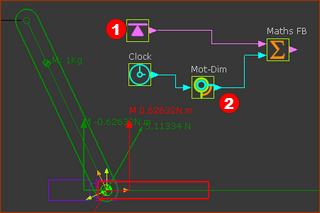We get Torque data from a Force-Data FB. We get Angular-Velocity data from a Motion-Dimension FB. Prepare the model: 1.Add a Rocker - see Tutorial 1 2.Edit the CAD-Line of the Rocker, to open the CAD-Line dialog-box. In Mass Properties tab > User Mass Properties . Enter a value for the Mass In Mass Properties tab > User Mass Properties . Enter X and Y values to move the Mass from the start-Point of the CAD-Line. We will not enter a value for the Inertia or Radius of Gyration. This makes the mass a Point Mass - the mass of the Rocker is reduced to a particle. 3.Add a Force-Data FB, open the Force-Data FB dialog-box, select the Pin-Joint, and select the Point in the Base-Part to find the Torque that acts-on the Base-Part.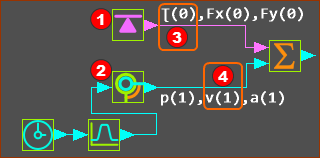See image above - the Wires and Data-Channels of the Force-Data FB and the Motion-Dimension FB Prepare the Math FB: 1.Open the Math FB dialog-box: 2.Add two Input-Connectors with the Add-Input button 3.Select Power for the Output Data Type. 4.Click UPDATE button after we select the Output Data Type and before we add equations. 5.Enter the Equations to calculate Power. The Math FB uses SI units for all data at its input-connectors The equation in the Math FB is: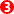×p(0)*v(1) Wire-Number 0 (top), Channel 1 (p = Torque) × Wire-Number 1 (bottom wire), Channel 2 (v = Angular Velocity)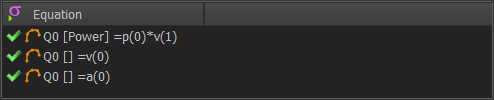MathFB- Power= Torque x Ang.Vel SYNTAX and VALID Equations: Click the Update after you edit the equation to find if its syntax is correct or not correct. For example, the syntax is not correct if it does not have the correct number of parentheses. The reason is given as a message in the Feedback-Area. The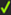at the left of each equation shows whether its syntax is correct.

### Input-Wires, Output Wires: Channels and Units

Each wire has three channels.

 ...wire from... Channel 1 Label & Units Channel 2 Label : Units Channel 3 Label & Units Data Type / Notes Linear Motion FB Machine Angle   Deg Machine Angular Velocity : Deg/sec Machine Angular Acceleration : Deg/sec/sec Note: Velocity: is a function of Machine Speed in Machine Settings dialog-box. Gearing FB Dis Input Unit Vel Unit/s Acc Unit/s/s Output Data is a function of input data type - Linear or Rotary Motion Dis mm or deg Vel mm/s or deg/s Acc mm/s/s or deg/s/s Output Data: motion-values, Linear or Rotary Change the Output Data Type to the match input Units Motion-Dimension Dis. mm or deg Vel. or Ang. Vel. mm/s or rad/s Acc mm/s/s or rad/s/s If Slider, Data-Type is Linear, If Rocker, Data-Type is Rotary Measurement Dis mm or deg Vel mm or deg Acceleration If Data-Type is Linear or Rotary, and depends on the Measurement. Point FB Displacement Velocity Acceleration Data Type is Linear. Cam-Data FB Pressure Angle   deg Contact Pressure Angel 1 deg Contact Pressure Angle 2 deg Radians Radius-of-Curvature Cam 1 Radius-of-Curvature Cam 2 ---- Linear Units Contact Force Total Contact Force, X component Contact Force, Y component Units as set in Machine Settings dialog-box. Shear Stress Cam 1 Shear Stress Cam 2 Units as set in Machine Settings dialog-box. Force Data FB Joint Total Force Force, X Component Y Component Units as set in Machine Settings dialog-box. Cam Contact Force X Component Y Component Units as set in Machine Settings dialog-box. Spring Force X Component Y Component Units as set in Machine Settings dialog-box. Math FB A 'special' : Any Unit As per equation As per equation You connect a wire to it and convert its units to a different Data Type. Beware of units.